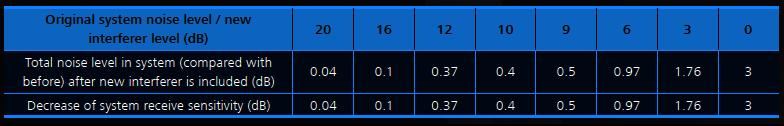TELETOPIX.ORG

Telecom Techniques Guide

LTE

# Analysis of Background Noise in LTE RF

Assume that the eNodeB receiver’s IF bandwidth is Bw (unit: MHz), and the eNodeB receive noise figure is Nf (unit: dB), The equivalent noise level of the eNodeB receiver is as follows:

No = –174 + 10 log (Bw) + Nf (Unit: dBm)

If the demodulation carrier-to-interference ratio C/I (unit: dB) of the eNodeB receive system for a particular modulation scheme (MCS), then the theoretical receive sensitivity of the eNodeB is as follows:

So = No + (C/I) m, where, (C/I) m is the minimum demodulation C/I.

The noise level directly affects the eNodeB receive sensitivity, that is, when the noise level rises by 1 dB, the eNodeB receive sensitivity decreases by 1 dB. In the system, 1 dB decrease in the receiver sensitivity of the system is regarded as the threshold of interference.

For LTE, sensitivity is calculated on per subcarrier rather than the entire channel allocation as in GSM, WCDMA or WiMAX because it is the basic bandwidth that needs to be demodulated by each UE. In actual component implementation level, receiver’s IF bandwidth and noise coefficient are affected by the specific circuits and can never reach the theoretical value or optimum value from a pure analog circuitry perspective.

Assume that the external receive intra-frequency spurious interference has the feature of the quasi-white noise, the influence of the interference to the system is that the interference adds to the original equivalent noise of the system and then raises the receive noise level of the system. BelowTable lists the receive background noise rise level due to the presence of the external interference at level specified.In general, the new total interference increase from original due to extra interferer can be represented by:

10.log(1+10^( P/10))

P = new interferer level compared to the original level in dB.

Assume that the original noise level of the system is 1 w:

1. The interference level is 0 dB lower than the original noise level of the system, that is, the interference level is also 1 w. The total noise level of the system is (1 + 1 = 2 w). Therefore, after the system is interfered, the total noise level increase is as follows: 10 log (2 w/1 w = 2) = 3 dB.
2. The interference level is 3 dB lower than the original noise level of the system, that is, the interference level is 0.5 times of the original noise level (1/103/10 = 0.5), that is, 0.5 w. The total noise level of the system is 1 + 0.5 = 1.5 w. Therefore, after the system is interfered, the total noise level increase is as follows: 10 log (1.5 w/1 w = 1.5) = 1.76 dB.
3. The other values are calculated in the similar way: As shown in the preceding table, when the original receive sensitivity of the system decreases by 0.4 dB, the allowed interference level must be 10 dB lower than the original receive noise level of the system. When the original receive sensitivity of the system decreases by 0.1 dB, the allowed interference level must be 16 dB lower than the original receive noise level of the system. When the interference level is equal to the original receive noise level of the system, the receive sensitivity of the system decreases by 3 dB.

In the broadband system, the allowed interference level is generally 6 dB lower than the original receive noise coefficient of the system. Therefore, the original receiver sensitivity of the system decreases by 1 dB.